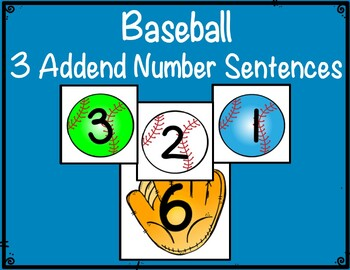Subject
Resource Type
File Type

PDF

(14 MB|35 pages)
Standards
Also included in:
1. Do you need some baseball themed literacy and math activities? These resources are for you! Easy Prep Baseball Bundle With Cross-Curricular Centers & Activities for Lower Elementary Children! This bundle includes both literacy and math resources for kindergarten and first grade students. Basebal
\$50.00
\$32.00
Save \$18.00
• Product Description
• StandardsNEW

Do you need a baseball themed math activity? This resource is for you! Baseball 3 Addend Number Sentence Math Center! Have your students practice their mental math and 3 addend addition and subtraction number sentences with this fun small group or center activity. Baseball fun for everyone!

Resource Prep: Print, laminate and cut all cards and pieces to the activity. Note: You can choose to do all the cards or take out the number of sentences you would like to work on. Copy how many worksheets you will need for your students.

Resource Includes the Following:

☆ 80 Baseball Game Card Sets: 0-20 Addition & Subtraction Facts

☆ Baseball Build an Addition Sentence Mat & Build a Subtraction Sentence Mat

☆ Baseball Build an Addition Sentence Worksheet & Build a Subtraction Sentence Worksheet

Resource Directions:

Baseball Build an Addition or Subtraction Number Sentence: Addition: Children begin with any of the baseballs to build their number sentence. Children must have each of the cards to make a number sentence. Ex: 1st addend blue baseball: 1; 2nd addend white baseball: 2; 3rd addend green baseball: 3; and then the sum Glove card: 6; 1 + 2 + 3 = 6 Children can stack the cards together.

Subtraction: Children begin with the Glove Card and to build their number sentence. Children must have each of the cards to make the number sentence: Ex: Minuend Glove Card: 6; 1st subtrahend white baseball: 2; 2nd subtrahend green baseball: 3; and then the difference blue baseball card: 1; (6-2)-3=1. Children can stack the cards together.

Baseball Build an Addition Number Sentence Mat & Build a Subtraction Number Sentence Mat: Children can use these mats to help organize how to make a number sentence. A good resource for students who are just learning number sentences or for those students who need visual aids.

Baseball Build an Addition Sentence Number Worksheet & Build a Subtraction Number Sentence Worksheet: Children can use these worksheets to record their work and as an assessment piece for teachers to see if students are understanding the concepts.

Kids have some baseball themed while building 3 addend number sentences!

✤ Please check out the preview for this product.

✤ Don’t forget to leave feedback. You will receive TPT credits that can be used on your future purchases!

Related Products

Baseball Math Centers

Baseball Literacy Centers

Spring Themed Literacy & Math Bundle

Spring Unit: Activities & Center

Fluently add and subtract within 20 using mental strategies. By end of Grade 2, know from memory all sums of two one-digit numbers.
Add and subtract within 20, demonstrating fluency for addition and subtraction within 10. Use strategies such as counting on; making ten (e.g., 8 + 6 = 8 + 2 + 4 = 10 + 4 = 14); decomposing a number leading to a ten (e.g., 13 - 4 = 13 - 3 - 1 = 10 - 1 = 9); using the relationship between addition and subtraction (e.g., knowing that 8 + 4 = 12, one knows 12 - 8 = 4); and creating equivalent but easier or known sums (e.g., adding 6 + 7 by creating the known equivalent 6 + 6 + 1 = 12 + 1 = 13).
Relate counting to addition and subtraction (e.g., by counting on 2 to add 2).
Understand subtraction as an unknown-addend problem. For example, subtract 10 – 8 by finding the number that makes 10 when added to 8.
Apply properties of operations as strategies to add and subtract. If 8 + 3 = 11 is known, then 3 + 8 = 11 is also known. (Commutative property of addition.) To add 2 + 6 + 4, the second two numbers can be added to make a ten, so 2 + 6 + 4 = 2 + 10 = 12. (Associative property of addition.)
Total Pages
35 pages
N/A
Teaching Duration
N/A
Report this Resource to TpT
Reported resources will be reviewed by our team. Report this resource to let us know if this resource violates TpT’s content guidelines.